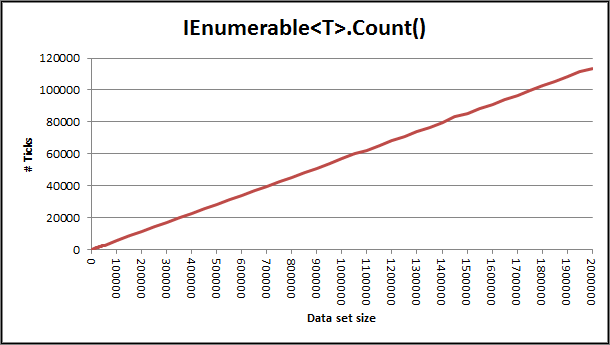# LINQ query performance

A while ago I was reviewing some code and I came across some code that looked like this

```if (corpus.Where(a => a.SomeProperty == someValue).Count() > 0)
{
// Do Stuff
}```

And it got me thinking that it may not be the best way to do this. What is really being asked here is: “Are there any items in the enumerable?” The count is not actually important in this situation. I considered that it would probably be more efficient to write:

```if (corpus.Where(a => a.SomeProperty == someValue).Any())
{
// Do stuff
}```

Then I read somewhere (unfortunately, I didn’t note the URL) that for certain situations the `.Any()` extension method on `IEnumerable<T>` can be inefficient in certain scenarios. For instance, if concrete type is actually a `List<T>` which maintains its own `Count`. In that instance the cost of setting up the `Enumerator` and calling `MoveNext()` to determine the existence of at least one element would be more expensive an operation than calling `Count` on the `List<T>`.

I was curious about that so I set about working out the relative performance characteristics of a number of the LINQ extension methods. I should note that these were all on LINQ to Objects out of the box so don’t measure how these methods would perform relatively for things like, say, LINQ to SQL.

I tested various scenarios, some where the `IEnumerable<T>` is a lightweight generator of elements, in this case an `Enumerable.Range(…)`, in other cases I used a `List<T>` either by a concrete reference or by an reference to the `IEnumerable<T>` interface.

All timings in this post relate to my desktop machine which is running Windows 7 64bit with 8Gb RAM and an AMD Phenom II X4 955 running at 1.6GHz (which for some unknown reason it won’t run at the full 3.2GHz)

### Counting elements

In the first set of tests I counted the number of elements. For the cases where I called the `Count` property directly on the `List<T>` and used the `Count()` extension method on `IEnumerable<T>` where the concrete type was the `List<T>` the result was returned in O(1). The LINQ method was 24 times slower.

Where the `IEnumerable<T>` did not also implement the `ICollection<T>` interface (as in the case where the values were being generated by `Enumerable.Range(…)` method) the `Count()` extension method took O(n) time to return the answer.The graph above shows the number of Ticks (vertical axis) taken to complete the counting task with n (horizontal axis) elements. A tick is roughly 1/1600th of a millisecond. So for 2000000 elements it took 72.5ms to count them.

Compare that for instances where the `Count` property was called directly (0.00413 Ticks or 0.00258µs [millionths of a second]) or where the `Count()` method was called on something that could be cast to an `ICollection` or `ICollection<T>` (0.0989 Ticks or 0.0618µs)

So far it looks good for cases where the underlying type implements the `ICollection<T>` or `ICollection` interface. However remember as soon as you start filtering the data (e.g. with a `Where()` method call) then you are returning an `IEnumerable<T>` which then operates in O(n) time. Also remember that the `Where()` clause will add some overhead as it has to process the filter as well.

### Any elements

It should be no surprise that using our test set of a `List<T>` and an `Enumerable.Range(…)` the `Any()` method runs in O(1) time. Both took similar amounts of time, the former taking 0.278 Ticks (0.174µs) per call, and the latter taking 0.296 Ticks (0.185µs) per call. I suspect that time on the latter is more due to the the small amount of additional time taken to generate additional elements as the enumerator progresses.

However, if you have a reference to something that already implements `ICollection<T>` which defines a `Count` property, such as a `List<T>`, you may find it is faster to perform `(corpus.Count>0)`. I found that for the `List<T>` I’d created for the test runs it was only marginally slower than the raw call to `Count` taking 0.00607 ticks (0.00379µs) per call.

### Any elements with filter

If you have a filter (a Where clause) then Any may take longer that O(1). It will take as long as it takes to find anything that matches the filter or O(n) if nothing matches the filter.

I ran three tests, one where the filtered condition was met on the first element, one where the condition was met in the middle of the set and one where the condition was not met until the last element.### Summary

If you have a concrete type the performance is better when using the `Count` property both for cases when you need to know the number of elements in the corpus or when you need to know if there any any elements at all.

If you simply need to know if there are any elements at all in the corpus then the use of `Any()` works out better than using the LINQ extension method `Count()` as `Count()` must traverse the entire corpus (unless it derives from `ICollection<T>` whereas `Any()` will short circuit at the first available opportunity.

## Published by Colin Mackay

I blog at ColinMackay.scot. I also talk at software development conferences.

## Join the Conversation

1.2.3.4 Comments

1.andre says:

Thanks for the post.
Did you try:
``` corpus.Any(a => a.SomeProperty == someValue) ```
It looks like it runs faster than using Where + Any for the quick tests I tried.

2.Frank says:

I have seen that many times LINQ is slower than the traditional loops and constructs but the ease of using them outweighs any performance drawbacks. Although, personally I use LINQ methods only on small to medium size data sets and for simple operations.

Leave a comment